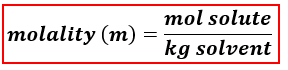# Problem: Determine the molality of an aqueous solution prepared by dissolving 0.112 moles of LiCl in 13.7 moles of H 2 O.A) 0.122 mB) 0.454 mC) 0.220 mD) 0.818 mE) 0.153 m

###### FREE Expert Solution
96% (160 ratings)
###### FREE Expert Solution

We’re being asked to calculate for the molality of an aqueous solution prepared by dissolving 0.112 moles of LiCl in 13.7 moles of H2O.

When calculating for molality, we use the following equation:Aqueous solution → water is the solvent

Solute → LiCl

The moles of the solute is already given.

We will calculate the molality of the solution using the following steps:

Step 1. Calculate the mass of the solvent (in kg).
Step 2. Calculate the molality of the solution.

Step 1. Calculate the mass of the water (in kg).

96% (160 ratings)###### Problem Details

Determine the molality of an aqueous solution prepared by dissolving 0.112 moles of LiCl in 13.7 moles of H 2 O.

A) 0.122 m

B) 0.454 m

C) 0.220 m

D) 0.818 m

E) 0.153 m

What scientific concept do you need to know in order to solve this problem?

Our tutors have indicated that to solve this problem you will need to apply the Molality concept. You can view video lessons to learn Molality. Or if you need more Molality practice, you can also practice Molality practice problems.

What is the difficulty of this problem?

Our tutors rated the difficulty ofDetermine the molality of an aqueous solution prepared by di...as medium difficulty.

How long does this problem take to solve?

Our expert Chemistry tutor, Dasha took 3 minutes and 2 seconds to solve this problem. You can follow their steps in the video explanation above.

What professor is this problem relevant for?

Based on our data, we think this problem is relevant for Professor Shakya's class at BC.